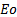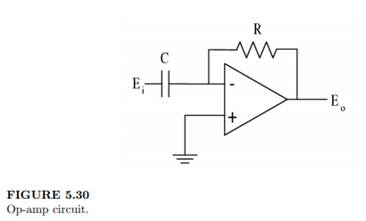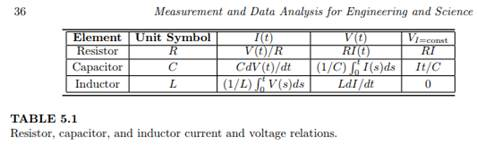### Create an Account

Home / Questions / Consider the operational amplifier circuit shown in Figure 5 30 and the information in Tab...

# Consider the operational amplifier circuit shown in Figure 5 30 and the information in Table 51 in which R is resistance C is capacitance I is current and t is time The transfer function of the

Consider the operational amplifier circuit shown in Figure 5.30 and the information in Table 5.1, in which R is resistance, C is capacitance, I is current, and t is time. The transfer function of the circuit can be written in the form where the output voltage,, equals a function of the input voltage, Ei , and other variables. (a) List all of the other variables that would be in the transfer function expression. (b) Using Kirchhoff’s laws, derive the actual transfer function expression. Identify any loops or nodes considered when applying Kirchhoff’s laws.Jul 26 2020 View more View LessSubscribe To Get Solution